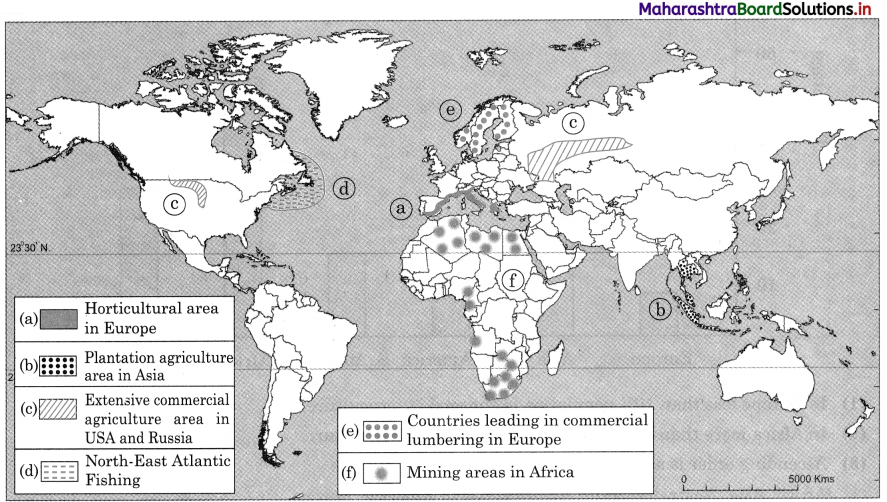# Maharashtra Board Class 12 Geography Important Questions Chapter 4 Primary Economic Activities

Balbharti Maharashtra State Board Class 12 Geography Important Questions Chapter 4 Primary Economic Activities Important Questions and Answers.

## Maharashtra State Board 12th Geography Important Questions Chapter 4 Primary Economic Activities

Identify the correct correlation.

A: Assertion R : Reasoning
Question 1.
A – Collection of forest products is not done on large scale in the equatorial forest region.
R – Humid and unhealthy climate of the equatorial region.
(a) Only A is correct.
(b) Only R is correct.
(c) Both A and R are correct and R is the correct explanation of A.
(d) Both A and R are correct but R is not the correct explanation of A.
(c) Both A and R are correct and R is the correct explanation of A.Question 2.
A – Commercial lumbering is not done in equatorial rainforest.
R – Trees in equatorial rain forest have hardwood.
(a) Only A is correct.
(b) Only R is correct.
(c) Both A and R are correct and R is the correct explanation of A.
(d) Both A and R are correct but R is not the correct explanation of A.
(c) Both A and R are correct and R is the correct explanation of A.

Question 3.
A – Lumbering is mostly practiced in coniferous forest region.
R – Coniferous forest are not very dense.
(a) Only A is correct.
(b) Only R is correct.
(c) Both A and R are correct and R is the correct explanation of A.
(d) Both A and R are correct but R is not the correct explanation of A.
(a) Only A is correct.

Question 4.
A – Fishing on the coastal areas of Japan is well developed.
R – Presence of cold and warm ocean water currents.
(a) Only A is correct.
(b) Only R is correct.
(c) Both A and R are correct and R is the correct explanation of A.
(d) Both A and R are correct but R is not the correct explanation of A.
(c) Both A and R are correct and R is the correct explanation of A.Question 5.
A – Animal husbandry has developed on a commercial basis in North America and Australia.
R – In North and South America animal husbandry is carried out with the help of advanced technology on a commercial basis.
(a) Only A is correct.
(b) Only R is correct.
(c) Both A and R are correct and R is the correct explanation of A.
(d) Both A and R are correct but R is not the correct explanation of A.
(c) Both A and R are correct and R is the correct explanation of A.

Identify the incorrect factor

Question 1.
Commercial fishing is carried along the following coastlines.
(a) North-East Atlantic coastline
(b) North-West Atlantic coastline
(c) Eastern coastline of Africa
(d) North-West coastline of Pacific Ocean
(c) Eastern coastline of AfricaQuestion 2.
Agriculture is developed in the following continents.
(a) Antarctica
(b) Asia
(c) Europe
(d) North America
(a) Antarctica

Question 3.
Animal husbandry is developed on a commercial basis in the following continents.
(a) North America
(b) Australia
(c) Asia
(d) South America
(c) Asia

Question 4.
The following physical factors are important for agriculture.
(a) Climate
(b) Transport
(c) Soil
(d) Relief
(b) TransportQuestion 5.
The following economic factors are essential for agriculture.
(a) Transport
(b) Storage facilities
(c) Climate
(d) Capital